# Physics 2102 Jonathan Dowling Physics 2102 Lecture 6

• Slides: 13Physics 2102 Jonathan Dowling Physics 2102 Lecture 6 Electric Potential IIExample Positive and negative charges of equal magnitude Q are held in a circle of radius R. 1. What is the electric potential at the center of each circle? • VA = • VB = • VC = 2. Draw an arrow representing the approximate direction of the electric field at the center of each circle. 3. Which system has the highest electric potential energy? UB –Q +Q A B C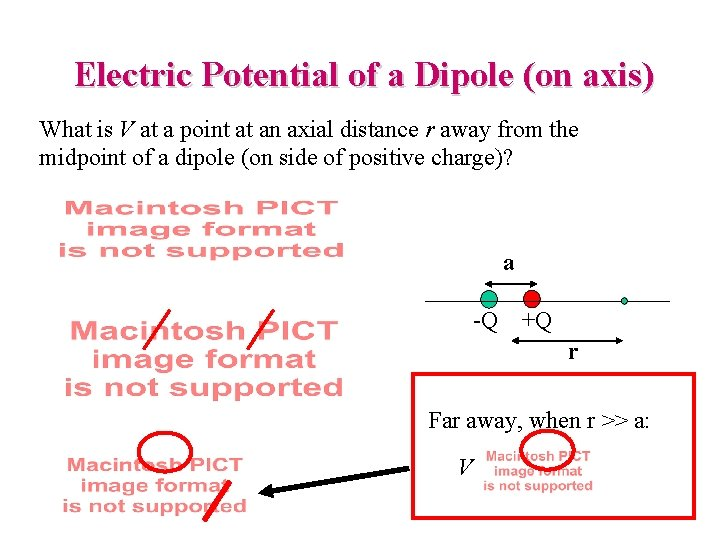Electric Potential of a Dipole (on axis) What is V at a point at an axial distance r away from the midpoint of a dipole (on side of positive charge)? a -Q +Q r Far away, when r >> a: VElectric Potential on Perpendicular Bisector of Dipole You bring a charge of Qo = – 3 C from infinity to a point P on the perpendicular bisector of a dipole as shown. Is the work that you do: a) Positive? b) Negative? c) Zero? U= Qo. V=Qo(–Q/d+Q/d)=0 a -Q +Q d P -3 C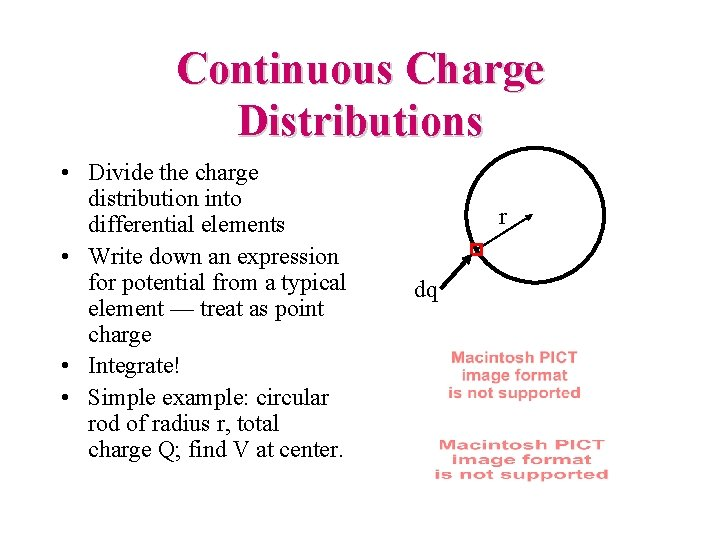Continuous Charge Distributions • Divide the charge distribution into differential elements • Write down an expression for potential from a typical element — treat as point charge • Integrate! • Simple example: circular rod of radius r, total charge Q; find V at center. r dq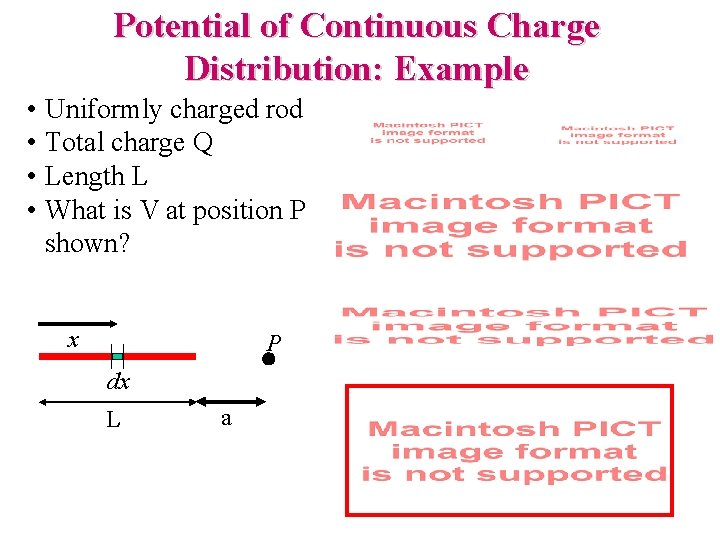Potential of Continuous Charge Distribution: Example • Uniformly charged rod • Total charge Q • Length L • What is V at position P shown? x P dx L a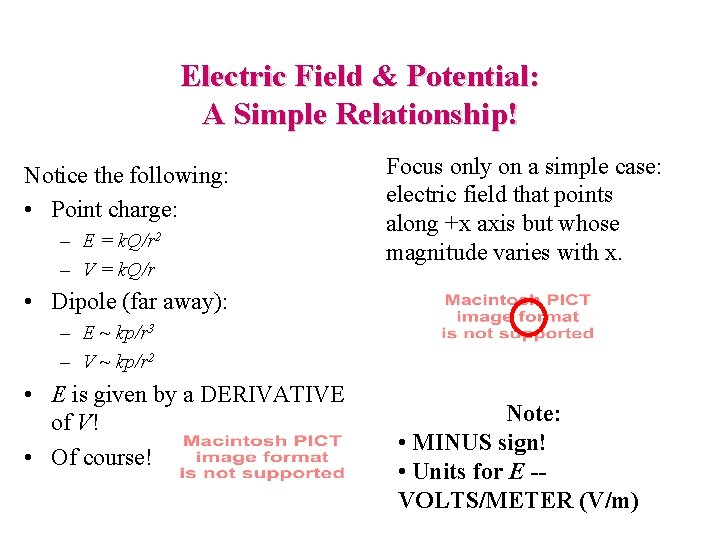Electric Field & Potential: A Simple Relationship! Notice the following: • Point charge: – E = k. Q/r 2 – V = k. Q/r Focus only on a simple case: electric field that points along +x axis but whose magnitude varies with x. • Dipole (far away): – E ~ kp/r 3 – V ~ kp/r 2 • E is given by a DERIVATIVE of V! • Of course! Note: • MINUS sign! • Units for E -VOLTS/METER (V/m)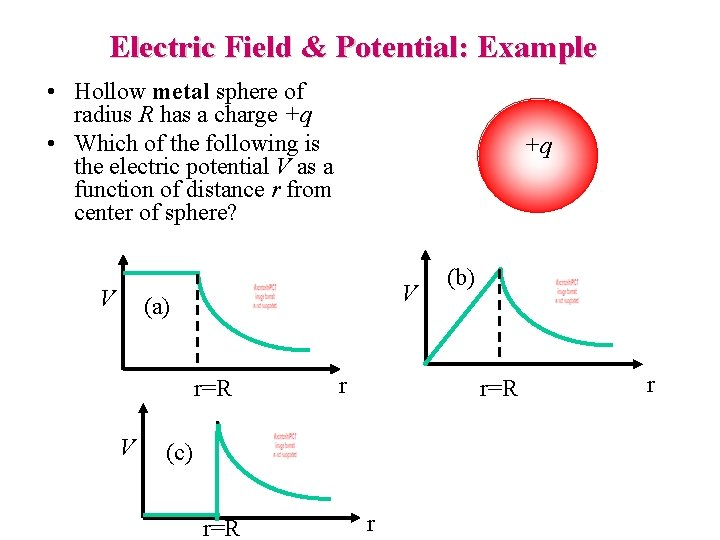Electric Field & Potential: Example • Hollow metal sphere of radius R has a charge +q • Which of the following is the electric potential V as a function of distance r from center of sphere? V +q V (a) r=R V r r=R (c) r=R (b) r r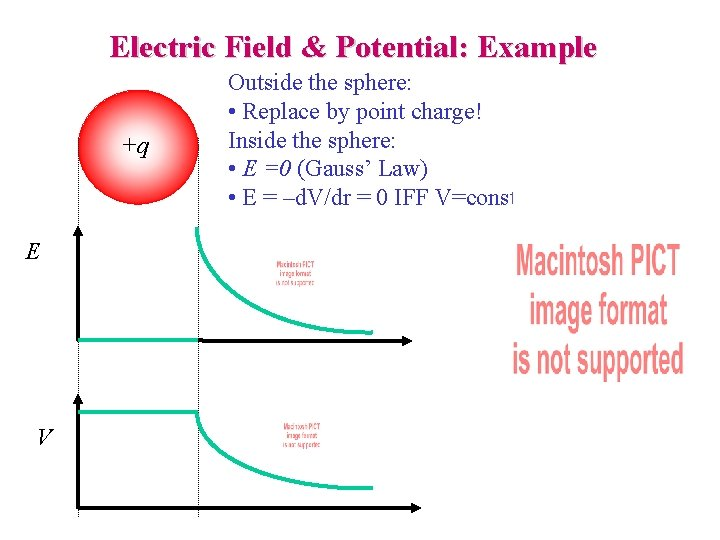Electric Field & Potential: Example +q E V Outside the sphere: • Replace by point charge! Inside the sphere: • E =0 (Gauss’ Law) • E = –d. V/dr = 0 IFF V=constantEquipotentials and Conductors • Conducting surfaces are EQUIPOTENTIALs • At surface of conductor, E is normal to surface • Hence, no work needed to move a charge from one point on a conductor surface to another • Equipotentials are normal to E, so they follow the shape of the conductor near the surface.Conductors change the field around them! An uncharged conductor: A uniform electric field: An uncharged conductor in the initially uniform electric field: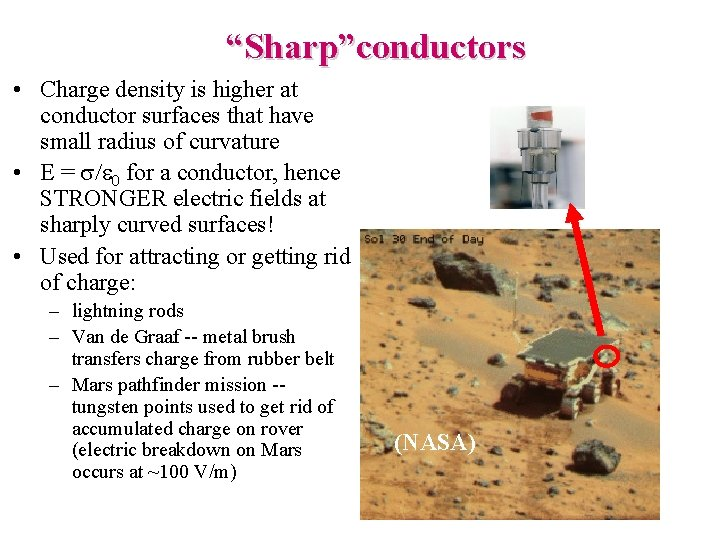“Sharp”conductors • Charge density is higher at conductor surfaces that have small radius of curvature • E = s/e 0 for a conductor, hence STRONGER electric fields at sharply curved surfaces! • Used for attracting or getting rid of charge: – lightning rods – Van de Graaf -- metal brush transfers charge from rubber belt – Mars pathfinder mission -- tungsten points used to get rid of accumulated charge on rover (electric breakdown on Mars occurs at ~100 V/m) (NASA)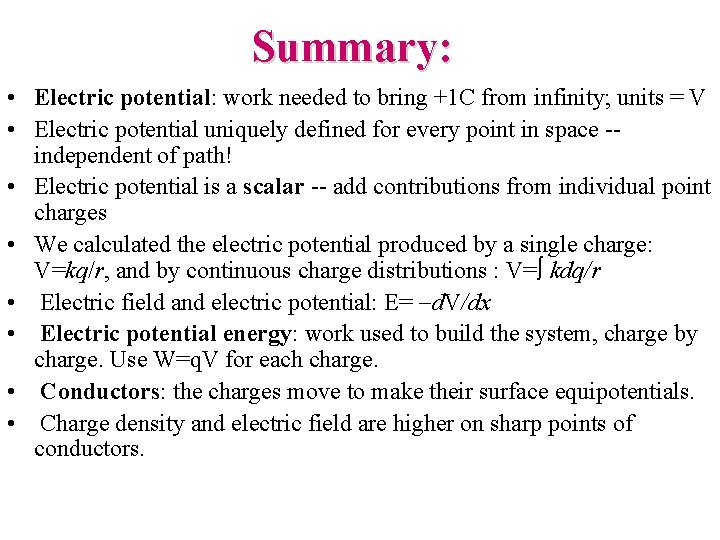Summary: • Electric potential: work needed to bring +1 C from infinity; units = V • Electric potential uniquely defined for every point in space -- independent of path! • Electric potential is a scalar -- add contributions from individual point charges • We calculated the electric potential produced by a single charge: V=kq/r, and by continuous charge distributions : V= kdq/r • Electric field and electric potential: E= -d. V/dx • Electric potential energy: work used to build the system, charge by charge. Use W=q. V for each charge. • Conductors: the charges move to make their surface equipotentials. • Charge density and electric field are higher on sharp points of conductors.